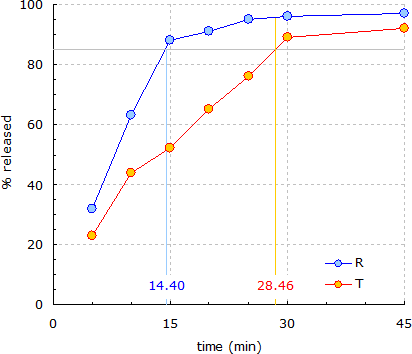## Sample after >85% release [Dissolution / BCS / IVIVC]

Hi Scopy,

I’m not sure which approach is the ‘correct’ one.

 t      R      T     5     32     23    10     63     44    14.40  85     51.04 15     88     52    20     91     65    25     95     76    28.46  95.69  85    30     96     89    45     97     92   

Highlighted rows at linear interpolated times for 85% release of R and T, respectively.

FDA (1st time >85% for T at 30 min, one sample after at 45 min): ƒ1 21.53, ƒ2 34.73
FDA (1st time >85% for T at 28.46 min, one sample after at 30 min): ƒ1 24.95, ƒ2 33.16
WHO (1st time >85% for R at 15 min, one sample after at 20 min): ƒ1 32.85, ƒ2 30.47
WHO (1st time >85% for R at 14.40 min, one sample after at 15 min): ƒ1 34.97, ƒ2 30.19

Quoting the FDA’s guidance:

For curves to be considered similar, ƒ1 values should be close to 0, and ƒ2 values should be close to 100. Generally, ƒ1 values up to 15 (0–15) and ƒ2 values greater than 50 (50–100) ensure sameness or equivalence of the two curves […]Can you elaborate how you arrived in this post at an ƒ2 of 99.5? Just by looking at the curves such a high ƒ2 does not seem to be possible.

 R   T   Δ    Δ2 32  23   9    81 63  44  19   361 88  52  36  1296 91  65  26   676 95  76  19   361 96  89   7    49 97  92   5    25          Σ  2849          n     7

My calculation by including all data showing intermediate results. Note that I simplified the original equa­tion to get rid of the stupid nested brackets:

$$\small{\\ f_2=50\cdot \log_{10}\left \{ \left [ 1+1/n\sum_{i=1}^{i=n}(R_i-T_i)^2\right ]^{-0.5}\cdot 100\right \}=\\ =50\cdot \log_{10}\left ( 100 / \sqrt{1+\sum_{i=1}^{i=n}(R_i-T_i)^2 / n}\right )=\\ =50\cdot \log_{10}\left ( 100 / \sqrt{1+2849 / 7}\right )=50\cdot \log_{10} \left (100/\sqrt {408} \right)=\\ =50\cdot \log_{10} (100/20.199)=50\cdot \log_{10} 4.9507=\\ =50\cdot 0.69467=34.73\; \; \; \; \blacksquare}$$

Dif-tor heh smusma 🖖Helmut SchützThe quality of responses received is directly proportional to the quality of the question asked. 🚮
Science QuotesIng. Helmut Schütz